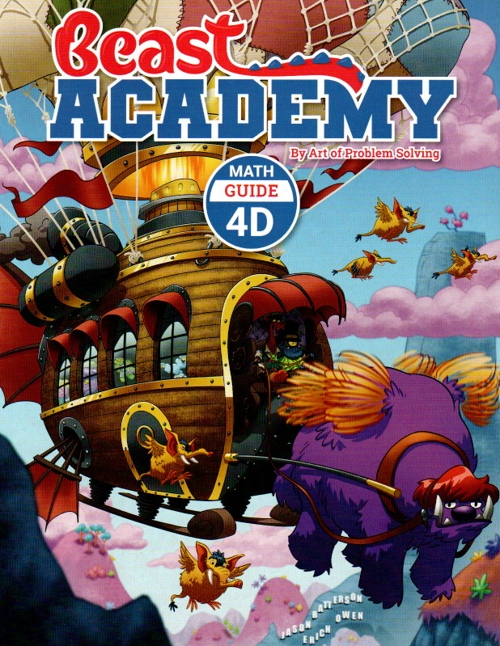Art of Problem Solving Beast Academy 4D Guide and Practice \$30 : Fractions : Multiplying fractions and mixed numbers and division by unit fractions. Decimals : Place value, converting between fractions and decimals, comparing and ordering decimals. Probability : Counting strategies review, coins, dice, random events, and geometric probability.